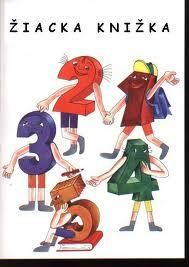# Student's 3366

Dorothy had a total of 22 scores in her school book. She had three times less score 1 than score 3. She had only two scores 2. How many scores, 1 and 3, did Dorothy have in her school book?

a =  5
b =  15

### Step-by-step explanation:

a+b = 22-2
a=b/3

a+b = 20
3a-b = 0

Pivot: Row 1 ↔ Row 2
3a-b = 0
a+b = 20

Row 2 - 1/3 · Row 1 → Row 2
3a-b = 0
1.33b = 20

b = 20/1.33333333 = 15
a = 0+b/3 = 0+15/3 = 5

a = 5
b = 15

Our linear equations calculator calculates it.Did you find an error or inaccuracy? Feel free to write us. Thank you!

Tips for related online calculators
Do you have a linear equation or system of equations and looking for its solution? Or do you have a quadratic equation?